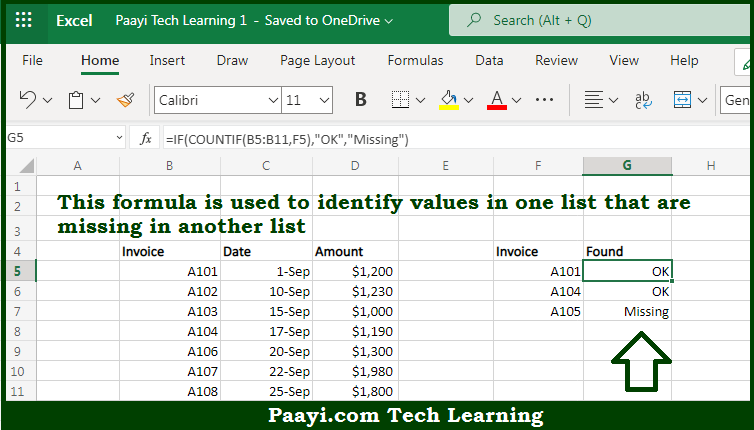# Learn How to Find Missing Values in Microsoft Excel

Written by | 0 Comments | 569 Views

In this article, you will learn how to evaluate things based on the LOOKUP function in Microsoft Excel using a single/combination(s) of functions. You will also know to Find Missing Values and see the generic formula.

Learn How to Find Missing Values in Microsoft Excel

The main purpose of this formula is to identify values in one list that are missing in another list. Here we will learn how to find the missing values in the given data range in the workbook in Microsoft Excel. That implies, with the help of a formula based on the COUNTIF, and IF functions you can able to identify values in one list that are missing in another list. So, with the help of this formula, you can able to find the missing values in the given data range in the workbook in Microsoft Excel.

General Formula to Find Missing Values

=IF(COUNTIF(list,value),"OK","Missing")

The Explanation to Find Missing ValuesSo we know that with the help of the given formula above you can able to identify values in one list that are missing in another list. Here we will learn how to find the missing values in the given data range in the workbook in Microsoft Excel. As we know that the COUNTIF function counts cells that meet the given criteria. It returns the number of occurrences found. If no cells meet the criteria, COUNTIF returns zero. You can use behavior directly inside an IF statement to mark values that have a zero count. So now you have learned how to find the missing values in the given data range in the workbook in Microsoft Excel.